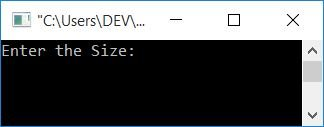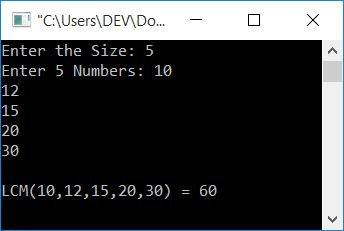# C program to find the LCM of n numbers

In this article, you will learn and get code for finding the LCM of n numbers. Or you can say that, here, the program is created to find the LCM of an array. However, before you begin the program, I recommend that you first visit Find LCM of Two Numbers (if you haven't already). Because the program given here is related to the program in that article.

## In C, find the LCM of n numbers

The question is: write a program in C to find out the LCM of n numbers in a given array. The answer to this question is:

```#include<stdio.h>
#include<conio.h>
int main()
{
int arr, n, mp, i, count;
printf("Enter the Size: ");
scanf("%d", &n);
printf("Enter %d Numbers: ", n);
for(i=0; i<n; i++)
scanf("%d", &arr[i]);
i=0;
mp = arr[i];
while(i<n)
{
if(mp<arr[i])
mp = arr[i];
i++;
}
while(1)
{
i=0;
count=0;
while(i<n)
{
if(mp%arr[i]==0)
count++;
i++;
}
if(count==n)
break;
else
mp++;
}
printf("\nLCM(");
for(i=0; i<(n-1); i++)
printf("%d,", arr[i]);
printf("%d) = %d", arr[i], mp);
getch();
return 0;
}```

This program was built and runs in the Code::Blocks IDE. Here is its sample run:Now supply 5 as the size and enter 5 numbers, say 10, 12, 15, 20, and 30. Then press the ENTER key to see the following output:#### Program Explained

The statement:

```while(i<n)
{
if(mp%arr[i]==0)
count++;
i++;
}```

is used to check whether the value at mp is divisible by all the numbers or not. If it is divisible by any number, then increment the value of count. After exiting this loop, check whether count is equal to the array size or not. If it is equal, then mp is divisible by all the numbers. Because every time the condition of if blocks evaluates to true and program flow goes inside the block, it increments the value of count.

For example, let's suppose the size of the array is 5 and the elements are 10, 12, 15, 20, and 30. The program then proceeds as follows:

• The element at index 0 (the first number) is set to mp.
• It is compared to the element of the next index (second number).
• If the value of mp is less than the number at the next index, then initialize its value to mp.
• This block of code is created only to assign the largest element of the array to mp, to start from the highest number.
• Using 1 as the while loop's condition, it always evaluates to true.
• Therefore, this loop will continue running until the break keyword occurs.
• Inside the loop, 0 is initialized to count, and i
• Create a while loop to run a certain number of times (5 times here).
• Inside the loop, check whether the value at mp is divisible by the number at the current index or not.
• If it is, then increment the value of count and go for the next element.
• After checking for all five elements
• Check whether the count is equal to the size of the array or not.
• If it is equal, then it means that the condition mp%arr[i]==0 evaluates to true 5 times. And it means that "mp" is divisible by all 5 numbers.
• Therefore, use the break statement and exit from the loop.
• And print its value as the LCM of the 5 numbers given.
• And if it is not equal, then increment the value of mp and continue the same process.
• Continue until it is divisible by all five numbers.

C Quiz

« Previous Program Next Program »